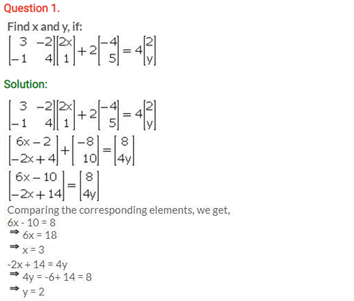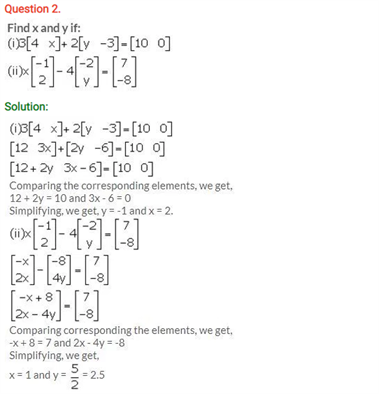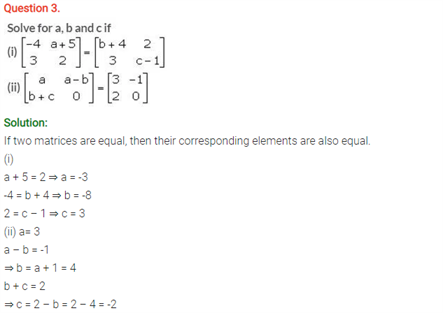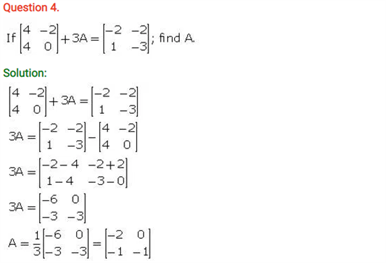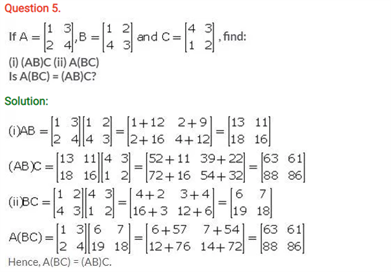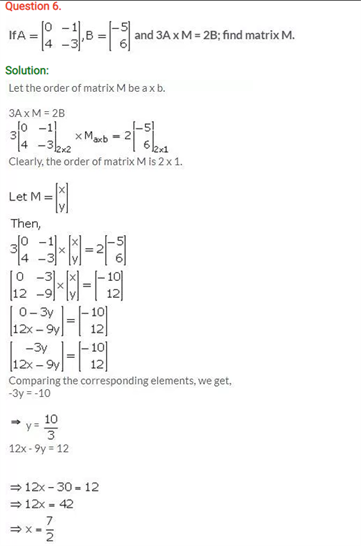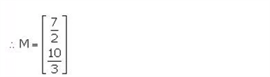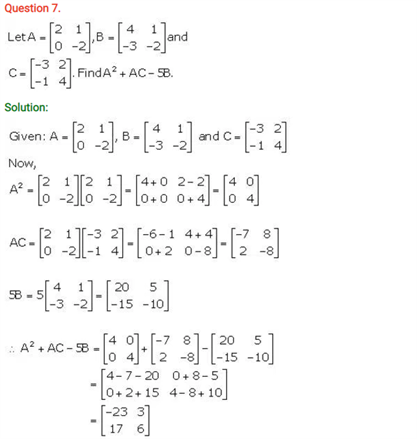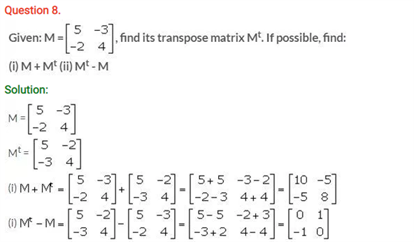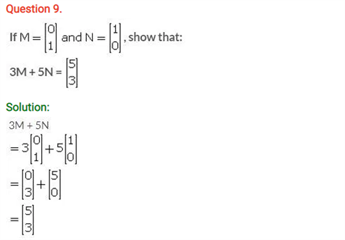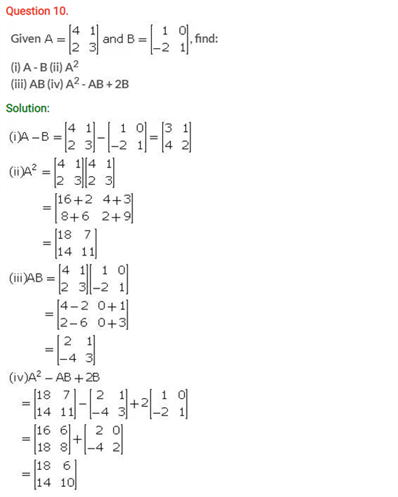# Selina Concise Maths Solution for ICSE Class 10 Chapter 9 Matrices

## Selina Class 10 Maths Solutions for Chapter 9

Here students will find some more questions on the Remainder and Factor Theorems. Students can find Important concepts on the ICSE Selina Solutions For Class 10 Maths. For the need to understand the concepts in a better way, lets us see the most basic definitions.

### Explanation:

The remainder theorem tells us that for any polynomial f(x), if you divide it by the binomial x−a, the remainder is equal to the value of f(a). The factor theorem tells us that if a is a zero of a polynomial f(x), then (x−a) is a factor of f(x), and vice-versa.

ICSE Solutions For Class 10 Maths brings you the excitement of solving all the important questions of this chapter. A matrice is the arrangement of collected numbers in fixed rows and columns. Matrices can contain real numbers and complex numbers as well. Lets us suppose, a 3 X 3 matrix is a matrix with 3 rows and 3 columns. Each number of the matrix is known as an element. Various operations can be performed in a matrix and there are various formulas for different operations. For the need to understand the concepts in a better way, lets us see the questions.

You will find the Class 10 Maths ICSE Solutions Chapter 9 Matrices below: